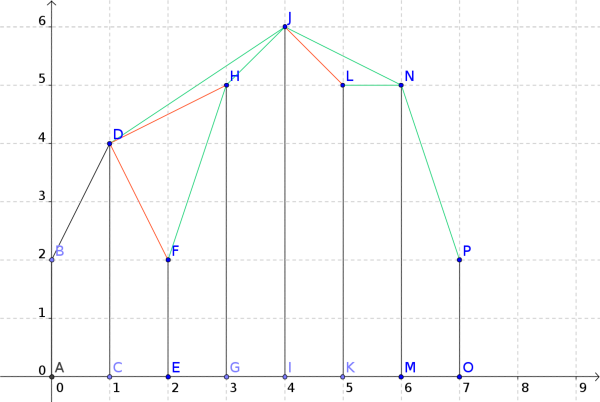# PAPARAZI - Editorial 2

Author: Yuriy Fedorov
Tester: Felipe Mota
Editorialist: Alei Reyes

EASY

# PREREQUISITES:

Vectors, Convex-Hull

# PROBLEM:

There are N vertical segments, the i-th with endpoints (i,0), (i,h_i).

The segment j is vissible from segment i, if the segment (i, h_i), (j,h_j) does not intersect another vertical segment.

Find the pair (i,j) such that j is vissible from i, and |j-i| is maximum.

# QUICK EXPLANATION:

Build the convex hull of the points (i,h_i) from right to left (in decreasing order of i), the rightmost vissible point from i is given by the segment of the convex hull that starts at (i,h_i).

# EXPLANATION:

Let’s denote the top of the i-th segment by P_i = (i,h_i), and let nxt[i] be the maximum j such that j is vissible from i.

Let’s brute force each pair (i,j) and iterate over each i \lt k \lt j to determine if the vertical segment (k,0), (k,h_k) intersects (i,h_i), (j,h_j).

We can use vectors to check the intersection, the cross product allows us to determine if two segments are in clockwise or anti-clockwise by looking to the sign of (P_j - P_i) \times (P_k-P_i). It is also possible to use geometry and calculate the equation of the lines, and their intersection, however vectors make it easier. The complexity of this approach is O(N^3).

The plan to is optimize our solution by finding nxt[i] using the values of nxt[i+1],nxt[i+2], \ldots, nxt[N].

Let j=nxt[i] and k=nxt[j], note that we should always go in clockwise direction i.e (P_k-P_j) \times (P_j-P_i) \gt 0, otherwise it would be possible to see k from i (a contradiction).

Let’s draw a segment from each P_i to P_{nxt[i]} and look at the sequence of points H_i = (P_i, P_{nxt[i]}, P_{nxt[nxt[i]]}, ...). The idea is to reuse the information gathered in H_{i+1} to find nxt[i].For example in the above picture H_F = (F, H, J, N, P), the green lines represent the segments with endpoints P_i, P_{nxt[i]}.

To calculate nxt[i] we have to find the smallet j such that the points P_i, H_{i+1,j} and H_{i+1,j+1} are clockwise. For example, in the picture to find nxt[D] we have to look at the sequence H_F and discard F because DFH are not clockwise, similarly H is discarded and nxt[D]=J. The red lines represent the discarded segments.

If the previous algorithm is implemented naively you will get a quadratic complexity (subtask 2), however note that to determine nxt[i] we have to remove a preffix of H_{i+1}, that means each point is removed and added once, so the complexity is linear. Note that the sequence H_i is actually the upper-hull of the points P_i, \ldots ,P_N.

3 Likes

I solved this problem with min stack, keeping the line segments endpoints in the stack.

2 Likes

can you explain a bit more

i did the same approchLet’s say the top most element in our stack is this yellow line segment. Now the slope made using (3,h3) and (2,h2) will be lesser than the slope of yellow line (we are just checking values without taking signs). so we can safely remove the yellow line segment and add the segment (3,h3) - (1,h1).
So basically h3 should be greater than or equal to the point of the yellow line to remove the top most element. And if you don’t know min stack you can read about it on cp-algorithms.com
See my submission for more details. We have to traverse the array two times :- One while moving from left to right and other while moving from right to left (Because we are only checking for bigger slopes in our stack).